< > unit5ReadOnly - page 13 of 13 Six Sigma Six Sigma Training - 6 Sigma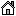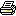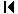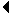13 of 13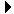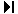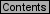8 9 10 11 12 13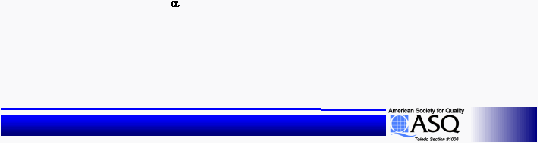Statistical Design of Experiments
Statistical Design of Experiments
Process Average
(Target) for:  A1  F2
= 0.90000 +/-
0.45631 (from 1.35631 to 0.44369)
T-bar
= 2.15
Mean
Mean
= A1
= A1
avg
avg
+ F2
+ F2
avg
avg
-
-
(2-1) * 2.15
(2-1) * 2.15
1.9 + 1.15 -
1.9 + 1.15 &#45;
-
2.15 = 0.9
2.15 = 0.9
CI
CI
=
=
Sq
Sq
Root ( F
Root ( F
= 0.05, 1, 5
= 0.05, 1, 5
* 0.084/2.667 ) = 0.456
* 0.084/2.667 ) = 0.456
n
n
eff
eff
=  8 / 1 + 1 + 1 = 8/3 = 2.667
=  8 / 1 + 1 + 1 = 8/3 = 2.667
F
F
.05, 1, 5
.05, 1, 5
= 6.61
= 6.61
Calculations
Calculations